# Solving Matrix Equations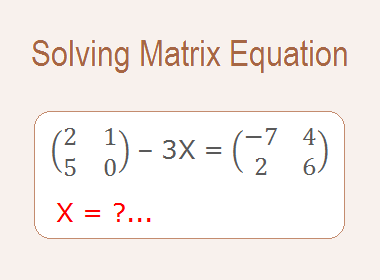## Solving Matrix Equations

While solving matrix equation A + X = B, where A and B are two given matrices of the same order and X is an unknown matrix, we proceed in a manner similar to the numbers.

********************

10 Math Problems officially announces the release of Quick Math Solver, an Android App on the Google Play Store for students around the world.

********************

Here, A + X = B

Adding the matrix (-A) to both sides of the matrix equation, we get -

(-A) + A + X = (-A) + B

or,    (-A + A) + X = B – A

or,    0 + X = B – A

or,    X = B – A,

which is the required solution of matrix equation A + X = B.

### Worked Out Examples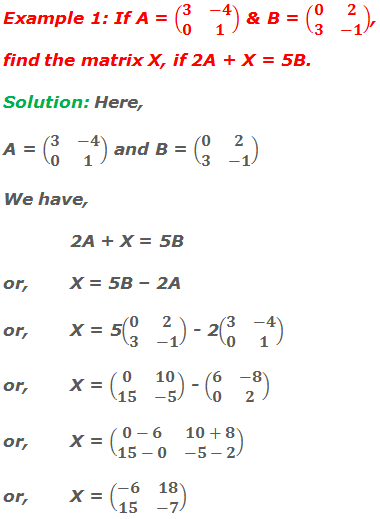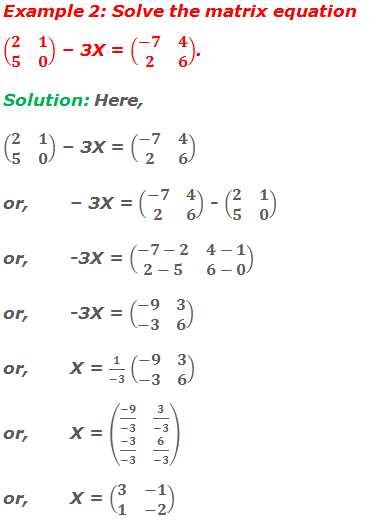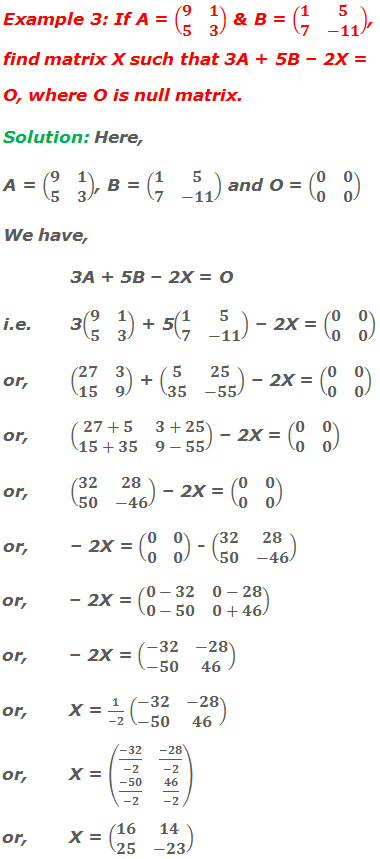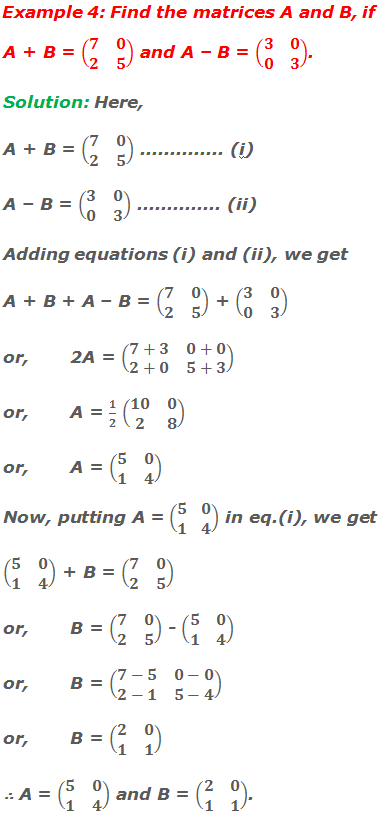#### Do you have any questions regarding the solution of matrix equations?

You can ask your questions or problems here, in the comment section below.